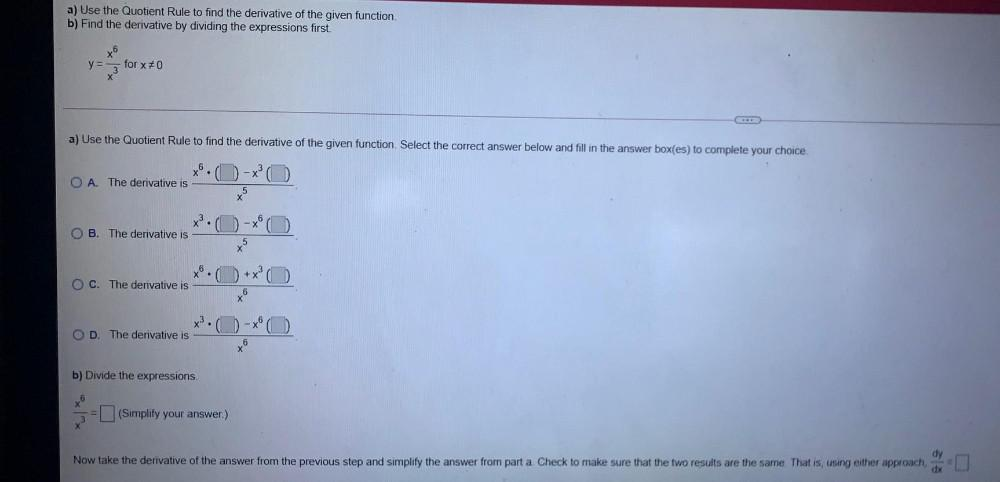Question:

# a) Use the Quotient Rule to find the derivative of the given function. b) Find the derivative by dividing the expressions first.a) Use the Quotient Rule to find the derivative of the given function. b) Find the derivative by dividing the expressions first. x6 for x70 y= X a) Use the Quotient Rule to find the derivative of the given function. Select the correct answer below and fill in the answer box(es) to complete your choice. O A. The derivative is 5 X B. The derivative is +x O C. The derivative is xo 6 X3. OD. The derivative is - (0) ta 6 b) Divide the expressions 6 (Simplify your answer.) dy Now take the derivative of the answer from the previous step and simplify the answer from part a Check to make sure that the two results are the same That is, using either approach, dx